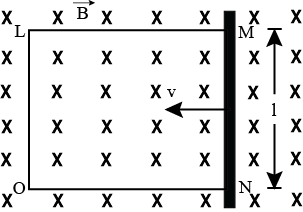Question

# A rectangular conductor LMNO is placed in a uniform magnetic field of 0.5T. The field is directed perpendicular to the plane of the conductor. When the arm MN of length of 20cm is moved towards left with a velocity of 10ms−1, calculate the emf induced in the arm. Given the resistance of the arm to be 5Ω, (assuming that other arms are of negligible resistance) find the value of the current in the arm.Solution

## Let ON be x at some instant. The emf induced in the loop =e. e=dϕdt=−d(Blx)dt e=Bl(−dxdt) =Blv= 0.5×0.2×10 =1V Current in the arm, 1=eR=15=0.2ASuggest corrections